# Transformer

Example of transformer with short circuit impedance, transmission resistances and load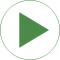# Information

This information is part of the Modelica Standard Library maintained by the Modelica Association.

This examples shows the operational behavior of transformer with short circuit impedance. The transformer is loaded with constant current magnitude of 250A but variable phase angle. The angle varies from 0 to 360 degrees within one second of simulation time.

Transformer data

• Nominal primary voltage `V1N = 1000 V`
• Nominal secondary voltage `V2N = 200 V`
• Nominal apparent power `SN = 50 kVA`
• Short circuit impedance `Zk = 0.72 Ohm + j*0.96 Ohm`
• Magnetizing current and core loss are not taken into account

Plot the real part of the secondary voltage `idealTransformer.v2.re` on the x axis and `idealTransformer.v2.im` on the y axis. The locus of this complex voltage `v2` is a circle. The center of the circle is the primary supply voltage divided by the transformation ratio of `n=5`. Since in this experiment the load current magnitude is constant, the voltage drop across the short circuit impedance of the transformer is constant, as well. The radius of the circle is equal to the constant magnitude of the voltage drop across the short circuit impedance.

# Components (15)

voltageSource idealTransformer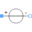Type: VoltageSource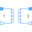Type: IdealTransformer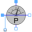Type: PowerSensor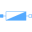Type: Impedance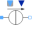Type: PowerSensor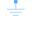Type: GroundType: Ground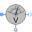Type: VoltageSensor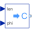Type: PolarToComplex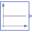Type: Constant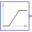Type: RampType: Constant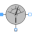Type: CurrentSensorType: CurrentSensor